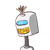# 1. Santosh requires 3 days more than Deepak to complete a work. If they wetogether, the work can be completed in 2 days. Fin

1. Santosh requires 3 days more than Deepak to complete a work. If they we
together, the work can be completed in 2 days. Find the number of day
required for each of them to complete the work.​

### 1 thought on “1. Santosh requires 3 days more than Deepak to complete a work. If they we<br />together, the work can be completed in 2 days. Fin”

1.Let Deepak complete a work in x day.

Santosh work be (x+3) days

therefore, work done by deepak in one day will be 1/x and work done by santosh be 1/x+3.

Work done by both of them in 2 days = 1/2

A.T.Q

1/x + 1/x+3 = 1/2

therefore by this we will get x=3 or x= -2

but the number of days is not negative

so we will take

x =3 and x+3 = 3+3=6

therefore deepak completes the work in 3 days and santosh in 6 days .